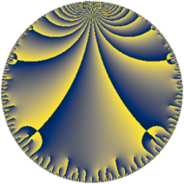# Properties

 Label 71.10.gLevel $71$ Weight $10$ Character orbit 71.g Rep. character $\chi_{71}(2,\cdot)$ Character field $\Q(\zeta_{35})$ Dimension $1272$ Newform subspaces $1$ Sturm bound $60$ Trace bound $0$

# Related objects

## Defining parameters

 Level: $$N$$ $$=$$ $$71$$ Weight: $$k$$ $$=$$ $$10$$ Character orbit: $$[\chi]$$ $$=$$ 71.g (of order $$35$$ and degree $$24$$) Character conductor: $$\operatorname{cond}(\chi)$$ $$=$$ $$71$$ Character field: $$\Q(\zeta_{35})$$ Newform subspaces: $$1$$ Sturm bound: $$60$$ Trace bound: $$0$$

## Dimensions

The following table gives the dimensions of various subspaces of $$M_{10}(71, [\chi])$$.

Total New Old
Modular forms 1320 1320 0
Cusp forms 1272 1272 0
Eisenstein series 48 48 0

## Trace form

 $$1272 q - 76 q^{2} - 395 q^{3} + 13542 q^{4} - 1610 q^{5} - 4334 q^{6} - 4827 q^{7} - 18627 q^{8} + 286810 q^{9} + O(q^{10})$$ $$1272 q - 76 q^{2} - 395 q^{3} + 13542 q^{4} - 1610 q^{5} - 4334 q^{6} - 4827 q^{7} - 18627 q^{8} + 286810 q^{9} - 53448 q^{10} - 170685 q^{11} + 138323 q^{12} - 93291 q^{13} + 985964 q^{14} - 966194 q^{15} + 4755334 q^{16} + 2558412 q^{17} - 2619769 q^{18} - 172269 q^{19} + 738120 q^{20} + 1797929 q^{21} + 3015622 q^{22} + 6635088 q^{23} + 4803145 q^{24} - 115100016 q^{25} + 19254293 q^{26} - 17686931 q^{27} + 15761532 q^{28} - 10052927 q^{29} + 62637435 q^{30} - 23748991 q^{31} + 10388192 q^{32} - 15097203 q^{33} + 33217854 q^{34} - 24823174 q^{35} + 27856812 q^{36} - 27156308 q^{37} - 43400577 q^{38} + 251072838 q^{39} - 129856160 q^{40} + 62087988 q^{41} - 91873950 q^{42} - 167299067 q^{43} + 60914131 q^{44} - 31536191 q^{45} - 50201490 q^{46} - 23317743 q^{47} + 10641329 q^{48} + 338745866 q^{49} + 303770229 q^{50} + 448039613 q^{51} - 979580293 q^{52} + 12085215 q^{53} - 182583505 q^{54} - 243892552 q^{55} + 30440435 q^{56} + 714581966 q^{57} - 244549660 q^{58} - 859013315 q^{59} - 1894499000 q^{60} - 876373921 q^{61} + 186553636 q^{62} - 196611021 q^{63} + 5528242635 q^{64} + 867018244 q^{65} + 2727701199 q^{66} - 286780089 q^{67} - 1689988351 q^{68} - 3073308182 q^{69} - 41489396 q^{70} - 2538053548 q^{71} - 6821388000 q^{72} + 128479023 q^{73} + 3282772334 q^{74} + 3407782757 q^{75} + 7133788844 q^{76} + 1087098389 q^{77} + 2715049934 q^{78} + 144243625 q^{79} - 7443877603 q^{80} - 4083269278 q^{81} - 10375744380 q^{82} + 1543773955 q^{83} - 4117054288 q^{84} + 7843382686 q^{85} + 3545751759 q^{86} + 5261609811 q^{87} - 818728797 q^{88} - 1369081479 q^{89} + 10041256259 q^{90} - 7299574322 q^{91} + 602797624 q^{92} - 2146129677 q^{93} - 683447510 q^{94} - 7007204038 q^{95} + 6601829126 q^{96} - 10081488030 q^{97} - 5942379880 q^{98} - 4074592265 q^{99} + O(q^{100})$$

## Decomposition of $$S_{10}^{\mathrm{new}}(71, [\chi])$$ into newform subspaces

Label Dim $A$ Field CM Traces $q$-expansion
$a_{2}$ $a_{3}$ $a_{5}$ $a_{7}$
71.10.g.a $1272$ $36.568$ None $$-76$$ $$-395$$ $$-1610$$ $$-4827$$